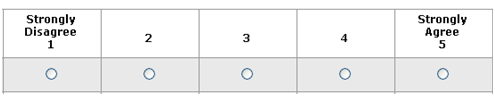# How To Interpret Survey Responses: 5 Techniques

Jeff Sauro, PhDClosed ended rating scale data is easy to summarize and hard to interpret.

Ideally you can compare the responses to an industry benchmark, a competitor or even a similar survey question from a prior survey. In most cases this data doesn’t exist, it’s too expensive or too difficult to obtain.

This leaves product managers and researchers to do their best in interpreting the raw responses.

For example, a recent survey I worked on asked a question about what users thought of the visual appeal of the software. Users were given a five point rating scale (from strongly disagree to strongly agree).Here are the responses from 18 users:

5, 5, 5, 5, 4, 5, 3, 4, 5, 5, 5, 5, 4, 5, 1, 2, 3, 4

Because the question was just written for the survey, there’s no historical or comparative data.

To find more meaning in this jumble of numbers, the first thing you need to do is compute the mean and standard deviation. While you won’t necessarily report them, you’ll need them for some of the subsequent steps.

There were 18 responses and the mean was a 4.167 and the standard deviation a 1.21. Here are five ways of making the raw responses more interpretable.

1. Percent Agree (78%): An old marketing trick is to summarize the percent of respondents who agreed to the item. There were 14 of the 18 respondents who chose a 4 or 5 (the Agree’s).
2. Top-Box (56%) or Top Two box (78%) scoring: For 5-point scales the top box is strongly agree, which generates a score of 56%. The top-two box score is the same as the agree score.
3. Net Top Box (50%): Count the number of respondents that select the top choice (strongly agree) and subtract the number that select the bottom choice (strongly Disagree choice). The popular Net Promoter Score uses a variation on this one (it subtracts the bottom six from the top 2 boxes). A Forrester annual report called the Customer Experience Index subtracts the top 2 bottom responses from the top-2 top responses (called the CxPi).
4. Z-Score to Percentile Rank (56%): This is a Six-Sigma technique. It converts the raw score into a normal score—because rating scale means often follow a normal or close to normal distribution. We just need a reasonable benchmark to compare the mean to. I’ve found that 80% of the number of points in a scale is a good place to start (a meta-analysis by Nielsen & Levy also found this). For a 5 point scale use a 4 (5*.80=4), for a 7 use 5.6 and for 11 use 8.8. Next follow these three steps.
1. Subtract the benchmark from the mean: 4.167-4 = .167
2. Divide the difference by the standard deviation: .167/1.21 = .1388. This is called a z-score (or normal score) and tells us how many standard deviations a score of 4.167 falls above or below the benchmark.
3. Convert the Z-score to a percentile rank: Using the properties of the normal curve we find out what percent of area falls below the .1388 standard deviations above the mean using a calculator or lookup table, we get .556 or 56%.
5. Coefficient of Variation (29%): The standard deviation is the most common way to express variability but it’s hard to interpret—especially when you use a mix of scales points (e.g. 5 and 7). The CV makes interpreting a bit easier by dividing the standard deviation by the mean (1.21/4.167 = .29). Higher values indicate higher variability. I’ve seen responses with similar means but with noticeably different coefficient of variations indicating respondents have inconsistent attitudes. The CV is a measure of variability, unlike the first four which are measures of the central tendency, so it can be used in addition to the other approaches.

As you can see, many of the methods generate reassuringly similar results. Here’s another example using 15 responses to a 7 point scale on perceived ease of use:

7, 5, 2, 3, 6, 1, 5, 7, 7, 6, 6, 6, 7, 7, 6

This generates a mean of 5.4 and a standard deviation of 1.92

I’ve summarized the results in the table below along with the results of the five point scale.

 5-Point Example 7-Point Example Percent Agree 78% 80% Top-2-Box 78% 67% Top-Box 56% 33% Net Top Box 50% 27% Z-Score to % 56% 46% CV 36% 29%

### Which is the best approach?

The “best” approach depends on the context and your situation. I’ve used all these at some point but I prefer the z-score approach for three reasons.

• It’s the only metric that includes variability in the score.
• It offers the most precision because it uses the mean.
• It tends to generate results in the middle of the others.

However, there are times when executive comprehension is more important than statistical precision. If you find it hard to explain the z-score approach and are unsure whether others will be comfortable with it, one of the other approaches will generate similar results (albeit less precisely).

The metrics are even more meaningful with confidence intervals, but that’s a topic for another blog. To help you get started, you can download an Excel file with the appropriate calculations for 5 and 7 point scales.

0
0